# Semicircle

Calculate the length of a semicircle with a radius of 6cm.

x =  18.8496 cm

### Step-by-step explanation: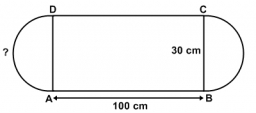Did you find an error or inaccuracy? Feel free to write us. Thank you!Tips to related online calculators
Need help to calculate sum, simplify or multiply fractions? Try our fraction calculator.

#### You need to know the following knowledge to solve this word math problem:

We encourage you to watch this tutorial video on this math problem:

## Related math problems and questions:Calculate the radius of the circle whose length is 107 cm larger than its diameter
• Circle - easy 2The circle has a radius 6 cm. Calculate:
• Central angleWhat is the length of the arc of a circle with a diameter of 46 cm, which belongs to a central angle of 30°?
• Wire D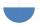Wire length 1 m is bent so that it forms a semicircle circuit (including the diameter). Determine the radius of the semicircle.Calculate the radius of the quadrant, which area is equal to area of circle with radius r = 15 cm.
• Quarter circularThe wire that is hooked around the perimeter of quarter-circular arc has length 3π+12. Determine the radius of circle arc.
• Hexagon in circleCalculate the radius of a circle whose length is 10 cm greater than the circumference of a regular hexagon inscribed in this circle.
• The length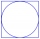The length of the circle is 24 cm. What is the length of the circular arc of the corresponding angle 30°?
• Circle r,DCalculate the diameter and radius of the circle if it has length 26.59 cm.
• Nine-gonCalculate the perimeter of a regular nonagon (9-gon) inscribed in a circle with a radius 13 cm.
• AnnulusThe radius of the larger circle is 8cm, the radius of smaller is 5cm. Calculate the contents of the annulus.
• n-gon IIWhat is the side length of the regular 5-gon circumscribed circle of radius 11 cm?
• Circular arcCalculate the length of the circular arc if the diameter d = 20cm and the angle alpha = 142 °
• The amphitheater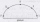The amphitheater has the shape of a semicircle, the spectators sit on the perimeter of the semicircle, the stage forms the diameter of the semicircle. Which of the spectators P, Q, R, S, T sees the stage at the greatest viewing angle?
• Rhombus and inscribed circleIt is given a rhombus with side a = 6 cm and the radius of the inscribed circle r = 2 cm. Calculate the length of its two diagonals.
• 6-gon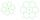Perimeter of regular hexagon is 113. Calculate its circumradius (radius of circumscribed circle).
• Circle and hexagon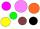Calculate the radius of a circle whose circumference is 8.4 cm longer than the inscribed regular hexagon's circumference.